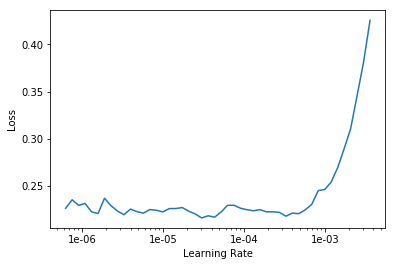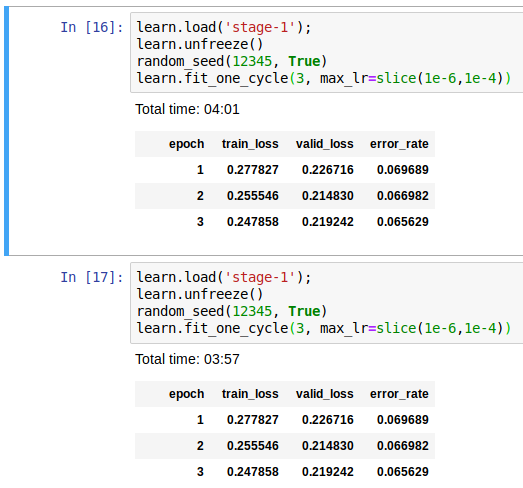# Lesson 1 - Part 1 V3 - Why is error rate different between runs with same data and learning rate?

I’m trying to understand if this behaviour is normal (because batches get selected randomly and therefore the error rate comes out different because of which batches happen to get selected during fit_one_cycle() ?)

Here is the gist of it:

learn = create_cnn(data, models.resnet34, metrics=error_rate)
learn.fit_one_cycle(4)

``````Total time: 01:39
epoch	train_loss	valid_loss	error_rate
1	1.384566	0.366372	0.119080
2	0.552150	0.286867	0.094723
3	0.343980	0.247791	0.087957
4	0.246434	0.236949	0.079161 <--- This is not great btw, I'm not sure why.
``````

==> Do the unfreeze and learn bit here… it’s much worse…

I do the LR finder bit and tweak the rate based on the Learning Rate plot belowlearn.unfreeze()
learn.fit_one_cycle(8, max_lr=slice(1.8e-6,1.5e-4))

``````Total time: 04:06
epoch	train_loss	valid_loss	error_rate
1	0.236310	0.233095	0.079161
2	0.214599	0.222202	0.074425
3	0.186991	0.226727	0.076455
4	0.156884	0.216776	0.073748
5	0.142581	0.215011	0.068336 <--- This is the best error rate I got
6	0.115727	0.221318	0.076455
7	0.107531	0.215099	0.073748
8	0.108737	0.212469	0.069689
``````

==> So I figured, I’d only train with 5 epochs with all else being same.

learn.unfreeze()
learn.fit_one_cycle(5, max_lr=slice(1.8e-6,1.5e-4))

``````Total time: 02:34
epoch	train_loss	valid_loss	error_rate
1	0.223285	0.226019	0.081191
2	0.210968	0.234650	0.075778
3	0.182657	0.217233	0.071042
4	0.149254	0.213546	0.073072
5	0.132440	0.216960	0.076455 <--- all these error rates are totally different now.. why?
``````

I’m confused as to why this is happening? Is this how it’s supposed to work?

1 Like

Did you save your model before calling load? I don’t see it.

Yes I did as “stage-1” … I just forgot to put it in the code sequence above as it’s not a screenshot. If I load the model and run lr-find on it, it shows the same graph as in the original notebook.

I read in a related thread that these issues may have something to do with the random seed being reset during fit_one_cycle but I haven’t tried it.

This is likely due to learning rate annealing. Basically, when using `fit_one_cycle`, your learning rate is changing behind the scenes over the course of the run.

Because of this, comparing the 5th epoch of `learn.fit_one_cycle(8)` is not equivalent to the last epoch of `learn.fit_one_cycle(5)`.

If you run them both with 5 or both with 8 epochs you should see more similar results.

If you want actual 100% identical runs, there are several random seeds and a few settings to set. There’s a `random_seed` function here that will do the trick:
I gave it a shot and